Archive
Special IssuesVolume 5, Issue 4, August 2016, Page: 108-112
The Problem of Countability of Highest Ordinals
Alexey Pavlovich Kulaichev, Department of System Analysis, Moscow State University, Moscow, Russia
Received: Mar. 16, 2016;       Accepted: Mar. 25, 2016;       Published: Jun. 28, 2016
Abstract
In this study we use the alternate point of view on the structure of ordinals, according to which each ordinal is the union of non-intersecting foregoing segments of ordinals of equal exponentiation. Each ordinal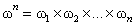is seen as the unionfor any j=1, n-1 instead traditional union of foregoing intersecting segments of ordinals of consistently increasing exponentiation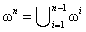. The first form corresponds to the geometric representation of ordinalas an infinite n-dimensional matrix. According traditional formulation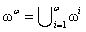, thus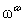is-countable union of countable ordinals sois countable. According to alternate formulation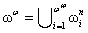for any n, thusis-union of ordinals and the findings will be different. These findings are: 1) the proof of countability of countable union of countable ordinals can not be directly or inductively transferred to its first limit-union; 2)seems to be the first uncountable ordinal with its power is equal to continuum; 3) the subsequent ascending degrees of-exponentiation of, i.e.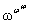,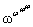,..., correspond to consecutive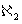,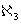,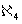, ... cardinals; 4) from here it also follows the direct justification of continuum hypothesis. Our study shows that in the domain of transfinite sets different points of view and its findings have the legal right to coexist as Nels Bohr's principle of complementarity in physics.
Keywords
Set Theory, Ordinals, Mathematical Induction, Absolute Truth
Alexey Pavlovich Kulaichev, The Problem of Countability of Highest Ordinals, Pure and Applied Mathematics Journal. Vol. 5, No. 4, 2016, pp. 108-112. doi: 10.11648/j.pamj.20160504.14
Reference

Georg Cantor, 1878, Ein beitrag zur mannigfaltigkeitslehre. Journal fr die reine und angewandte Mathematik, 84, 242-258.

Georg Cantor, 1883, Grundlagen einer allge. meinen Mannigfaltigkeitslehre: Ein mathematisch-philo-sophischer Versuch in der Lehre des Unendlichen. Leipzig: Teubner.

Deza, E., Deza, M., 2011, Figurate Numbers. World Scientific.

Felix Hausdorff, 1914, Grundzuge der Mengenlehre. Veit and Company, Leipzig.

Stephen Cole Kleene, 1967, Mathematical logic. N. Y.

Morris Kline, 1980, Mathematics. The loss of certainty. N. Y.

Kuratowski K., Mostowski A., 1967, Set theory. Amsterdam.

Lisi M., 2007, Some remarks on the Cantor pairing function. Le Matematiche, 62(1), 55-65.

Maydim A. Malkov, 2014, Stairs of Natural Set Theories, Pure and Applied Mathematics Journal. 3(3), 49-65.

Vereshchagin N. K., Shen A., 2002, Lectures on mathematical logic and theory of algorithms. Moscow, MCNME.